Select Page

# Definition

The quartile deviation is half the difference between the third quartile and the first quartile of a frequency distribution, or simply distribution. Mathematically, quartile deviation would be represented as follows;

Quartile deviation is also known as semi-interquartile range. Here, the difference between the third and first quartiles is called interquartile range. The interquartile range may be taken as measure of dispersion (i.e. the extent to which the values are spread out from the average).

## Characteristics of Quartile Deviation

i. The quartile deviation is simple to understand and easy to calculate.

ii. As a measure of variation, it is superior to the range because it is not affected by extreme values.

iii. The values of quartile deviation might be the same for two dissimilar distributions provided the quartiles are the same.

iv. It is not utilized in algebraic manipulation.

v. It is of the use only in a case where one wants to study the dispersion of items in the middle or the main body of the series. This usually happens in a frequency distribution where distribution tends to be intense in the middle or the main body of the series and the distribution of items towards the extremes are not of much significance.

### Example

We will find the semi-interquartile range or the quartile deviation for the distribution of weights of 120 students in following Table 19;

Table 19

 Weight (lb) Frequency (f) Class Boundaries Cumulative Frequency 110 – 119 1 109.5 – 119.5 0 120 – 129 4 119.5 – 129.5 5 130 – 139 17 129.5 – 139.5 22 140 – 149 28 139.5 – 149.5 50 150 – 159 25 149.5 – 159.5 75 160 – 169 18 159.5 – 169.5 93 170 – 179 13 169.5 – 179.5 106 180 – 189 6 179.5 – 189.5 112 190 – 199 5 189.5 – 199.5 117 200 – 209 2 195.5 – 209.5 119 210 – 219 1 209.5 – 219.5 120 ∑f = n = 120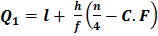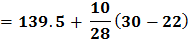Putting the values of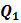and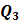in the formula, we get the following;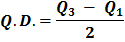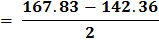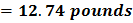## Explanation

We are aware of the fact that 50% of the values lie between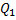and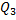. The range i.e. Median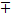Q.D, also contains approximately 50% of the values. In a symmetrical distribution, the quartile are equi-distant from the median and the quartile deviation measures the distance from the median to the lower quartile or the distance from the median to the upper quartile. Therefore, the in a symmetrical distribution, if we measure quartile deviation below and above the median than it will includes the central 50% of the values of the distribution. We always need to remember that, in a distribution where there is not complete symmetry, quartile deviation measures the average distance from the quartiles to the median.

Shares